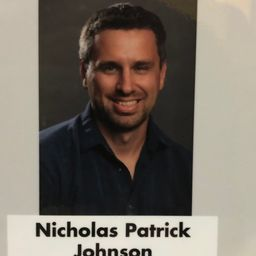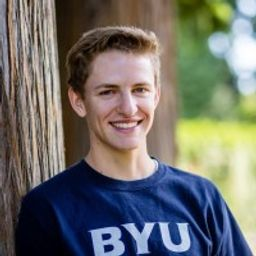Our Discord hit 10K members! 🎉 Meet students and ask top educators your questions.Join Here!

## Educators### Problem 1

Does 17 divide each of these numbers?
$$\begin{array}{llll}{\text { a) } 68} & {\text { b) } 84} & {\text { c) } 357} & {\text { d) } 1001}\end{array}$$James C.
Numerade Educator

### Problem 2

Prove that if $a$ is an integer other than $0,$ then
a) 1 divides $a . \quad$ b) $a$ divides 0 .James C.
Numerade Educator

### Problem 3

Prove that part (ii) of Theorem 1 is true.James C.
Numerade Educator

### Problem 4

Prove that part (iii) of Theorem 1 is true.James C.
Numerade Educator

### Problem 5

Show that if $a | b$ and $b | a,$ where $a$ and $b$ are integers, then $a=b$ or $a=-b$James C.
Numerade Educator

### Problem 6

Show that if $a, b, c,$ and $d$ are integers, where $a \neq 0,$ such that $a | c$ and $b | d,$ then $a b | c d .$James C.
Numerade Educator

### Problem 7

Show that if $a, b,$ and $c$ are integers, where $a \neq 0$ and $c \neq 0,$ such that $a c | b c,$ then $a | b$ .James C.
Numerade Educator

### Problem 8

Prove or disprove that if $a | b c,$ where $a, b,$ and $c$ are positive integers and $a \neq 0,$ then $a | b$ or $a | c .$James C.
Numerade Educator

### Problem 9

Prove that if $a$ and $b$ are integers and $a$ divides $b,$ then $a$ is odd or $b$ is even.James C.
Numerade Educator

### Problem 10

Prove that if $a$ and $b$ are nonzero integers, $a$ divides $b,$ and $a+b$ is odd, then $a$ is odd.James C.
Numerade Educator

### Problem 11

Prove that if $a$ is an integer that is not divisible by $3,$ then $(a+1)(a+2)$ is divisible by $3 .$James C.
Numerade Educator

### Problem 12

Prove that if $a$ is a positive integer, then 4 does not divide $a^{2}+2 .$James C.
Numerade Educator

### Problem 13

What are the quotient and remainder when
$$\begin{array}{l}{\text { a) } 19 \text { is divided by } 7 ?} & {\text { b) }-111 \text { is divided by } 11 ?} \\ {\text { c) } 789 \text { is divided by } 23 ?} & {\text { d) } 1001 \text { is divided by } 13 ?} \\ {\text { e) } 0 \text { is divided by } 19 ?} & {\text { f) } 3 \text { is divided by } 5 ?} \\ {\text { g) }-1 \text { is divided by } 3 ?} & {\text { h) } 4 \text { is divided by } 1 ?}\end{array}$$James C.
Numerade Educator

### Problem 14

What are the quotient and remainder when
a) 44 is divided by 8$?$
b) 777 is divided by 21$?$
c) $-123$ is divided by 19$?$
d) $-1$ is divided by 23$?$
e) $-2002$ is divided by 87$?$
f) 0 is divided by 17$?$
g) $1,234,567$ is divided by 1001$?$
h) $-100$ is divided by 101$?$Nick J.
Numerade Educator

### Problem 15

What time does a 12 -hour clock read
a) 80 hours after it reads $11 : 00 ?$
b) 40 hours before it reads $12 : 00 ?$
c) 100 hours after it reads $6 : 00 ?$James C.
Numerade Educator

### Problem 16

What time does a 24 -hour clock read
a) 100 hours after it reads $2 : 00 ?$
b) 45 hours before it reads $12 : 00 ?$
c) 168 hours after it reads $19 : 00 ?$James C.
Numerade Educator

### Problem 17

Suppose that $a$ and $b$ are integers, $a \equiv 4(\bmod 13),$ and $b \equiv 9(\bmod 13) .$ Find the integer $c$ with $0 \leq c \leq 12$ such that
a) $c \equiv 9 a(\bmod 13)$
b) $c \equiv 11 b(\bmod 13)$
c) $c \equiv a+b(\bmod 13)$
d) $c \equiv 2 a+3 b(\bmod 13)$
e) $c \equiv a^{2}+b^{2}(\bmod 13)$
f) $c \equiv a^{3}-b^{3}(\bmod 13)$James C.
Numerade Educator

### Problem 18

Suppose that $a$ and $b$ are integers, $a \equiv 11(\bmod 19),$ and $b \equiv 3(\bmod 19) .$ Find the integer $c$ with $0 \leq c \leq 18$ such that
a) $c \equiv 13 a(\bmod 19)$
b) $c \equiv 8 b(\bmod 19)$
c) $c \equiv a-b(\bmod 19)$
d) $c \equiv 7 a^{2}+3 b(\bmod 19)$
e) $c \equiv 2 a^{2}+3 b^{2}(\bmod 19)$
f) $c \equiv a^{3}+4 b^{3}(\bmod 19)$James C.
Numerade Educator

### Problem 19

Show that if $a$ and $d$ are positive integers, then $(-a)$ div $d=-a$ div $d$ if and only if $d$ divides $a$ .James C.
Numerade Educator

### Problem 20

Prove or disprove that if $a, b,$ and $d$ are integers with $d>0,$ then $(a+b)$ div $d=a \operatorname{div} d+b \operatorname{div} d$Mitchell C.
Numerade Educator

### Problem 21

Let $m$ be a positive integer. Show that $a \equiv b(\bmod m)$ if $a$ mod $m=b$ mod $m .$James C.
Numerade Educator

### Problem 22

Let $m$ be a positive integer. Show that $a$ mod $m=$ $b \bmod m$ if $a \equiv b(\bmod m)$James C.
Numerade Educator

### Problem 23

Show that if $n$ and $k$ are positive integers, then $\lceil n / k\rceil=$ $\lfloor(n-1) / k\rfloor+ 1$James C.
Numerade Educator

### Problem 24

Show that if $a$ is an integer and $d$ is an integer greater than $1,$ then the quotient and remainder obtained when $a$ is divided by $d$ are $\lfloor a / d\rfloor$ and $a-d\lfloor a / d\rfloor$ respectively.

Check back soon!

### Problem 25

Find a formula for the integer with smallest absolute value that is congruent to an integer $a$ modulo $m,$ where $m$ is a positive integer.James C.
Numerade Educator

### Problem 26

Evaluate these quantities.
$$\begin{array}{ll}{\text { a) }-17 \bmod 2} & {\text { b) } 144 \bmod 7} \\ {\text { c) }-101 \bmod 13} & {\text { d) } 199 \bmod 19}\end{array}$$James C.
Numerade Educator

### Problem 27

Evaluate these quantities.
$$\begin{array}{ll}{\text { a) } 13 \bmod 3} & {\text { b) }-97 \bmod 11} \\ {\text { c) } 155 \bmod 19} & {\text { d) }-221 \bmod 23}\end{array}$$James C.
Numerade Educator

### Problem 28

Find $a$ div $m$ and $a$ mod $m$ when
a) $a=-111, m=99$
b) $a=-9999, m=101$
c) $a=10299, m=999$
d) $a=123456, m=1001$

Check back soon!

### Problem 29

Find $a$ div $m$ and $a$ mod $m$ when
a) $a=228, m=119$
b) $a=9009, m=223$
c) $a=-10101, m=333$
d) $a=-765432, m=38271$James C.
Numerade Educator

### Problem 30

Find the integer $a$ such that
a) $a \equiv 43(\bmod 23)$ and $-22 \leq a \leq 0$
b) $a \equiv 17(\bmod 29)$ and $-14 \leq a \leq 14$
c) $a \equiv-11(\bmod 21)$ and $90 \leq a \leq 110$James C.
Numerade Educator

### Problem 31

Find the integer $a$ such that
a) $a \equiv-15(\bmod 27)$ and $-26 \leq a \leq 0$
b) $a \equiv 24(\bmod 31)$ and $-15 \leq a \leq 15$
c) $a \equiv 99(\bmod 41)$ and $100 \leq a \leq 140$James C.
Numerade Educator

### Problem 32

List five integers that are congruent to 4 modulo 12James C.
Numerade Educator

### Problem 33

List all integers between $-100$ and 100 that are congruent to $-1$ modulo $25 .$James C.
Numerade Educator

### Problem 34

Decide whether each of these integers is congruent to 3 modulo $7 .$
$$\begin{array}{ll}{\text { a) } 37} & {\text { b) } 66} \\ {\text { c) }-17} & {\text { d) }-67}\end{array}$$James C.
Numerade Educator

### Problem 35

Decide whether each of these integers is congruent to 5 modulo $17 .$
$\begin{array}{ll}{\text { a) } 80} & {\text { b) } 103} \\ {\text { c) }-29} & {\text { d) }-122}\end{array}$James C.
Numerade Educator

### Problem 36

Find each of these values.
a) $(177 \bmod 31+270 \bmod 31) \bmod 31$
b) $(177 \bmod 31 \cdot 270 \bmod 31) \bmod 31$James C.
Numerade Educator

### Problem 37

Find each of these values.
a) $(-133 \bmod 23+261 \bmod 23) \bmod 23$
b) $(457 \bmod 23 \cdot 182 \bmod 23) \bmod 23$James C.
Numerade Educator

### Problem 38

Find each of these values.
a) $\left(19^{2} \bmod 41\right) \bmod 9$
b) $\left(32^{3} \bmod 13\right)^{2} \bmod 11$
c) $\left(7^{3} \bmod 23\right)^{2} \bmod 31$
d) $\left(21^{2} \bmod 15\right)^{3} \bmod 22$James C.
Numerade Educator

### Problem 39

Find each of these values.
a) $\left(99^{2} \bmod 32\right)^{3} \bmod 15$
b) $\left(3^{4} \bmod 17\right)^{2} \bmod 11$
c) $\left(19^{3} \bmod 23\right)^{2} \bmod 31$
d) $\left(89^{3} \bmod 79\right)^{4} \bmod 26$James C.
Numerade Educator

### Problem 40

Show that if $a \equiv b(\bmod m)$ and $c \equiv d(\bmod m),$ where $a, b, c, d,$ and $m$ are integers with $m \geq 2,$ then $a-c \equiv$ $b-d(\bmod m)$James C.
Numerade Educator

### Problem 41

Show that if $n | m,$ where $n$ and $m$ are integers greater than $1,$ and if $a \equiv b(\bmod m),$ where $a$ and $b$ are integers, then $a \equiv b(\bmod n)$James C.
Numerade Educator

### Problem 42

Show that if $a, b, c,$ and $m$ are integers such that $m \geq 2$ $c>0,$ and $a \equiv b(\bmod m),$ then $a c \equiv b c(\bmod m c)$James C.
Numerade Educator

### Problem 43

Find counterexamples to each of these statements about congruences.
a) If $a c \equiv b c(\bmod m),$ where $a, b, c,$ and $m$ are integers with $m \geq 2,$ then $a \equiv b(\bmod m) .$
b) If $a \equiv b(\bmod m)$ and $c \equiv d(\bmod m),$ where $a, b, c, d,$ and $m$ are integers with $c$ and $d$ positive and $m \geq 2,$ then $a^{c} \equiv b^{d}(\bmod m) .$James C.
Numerade Educator

### Problem 44

Show that if $n$ is an integer then $n^{2} \equiv 0$ or 1$(\bmod 4)$James C.
Numerade Educator

### Problem 45

Use Exercise 44 to show that if $m$ is a positive integer of the form $4 k+3$ for some nonnegative integer $k,$ then $m$ is not the sum of the squares of two integers.James C.
Numerade Educator

### Problem 46

Prove that if $n$ is an odd positive integer, then $n^{2} \equiv$ $1(\bmod 8) .$James C.
Numerade Educator

### Problem 47

Show that if $a, b, k,$ and $m$ are integers such that $k \geq 1$ $m \geq 2,$ and $a \equiv b(\bmod m),$ then $a^{k} \equiv b^{k}(\bmod m)$James C.
Numerade Educator

### Problem 48

Show that $\mathbf{Z}_{m}$ with addition modulo $m,$ where $m \geq 2$ is an integer, satisfies the closure, associative, and commutative properties, 0 is an additive identity, and for every nonzero $a \in \mathbf{Z}_{m}, m-a$ is an inverse of $a$ modulo $m .$

Check back soon!

### Problem 49

Show that $\mathbf{Z}_{m}$ with multiplication modulo $m,$ where $m \geq$ 2 is an integer, satisfies the closure, associative, and commutativity properties, and 1 is a multiplicative identity.

Check back soon!

### Problem 50

Show that the distributive property of multiplication over addition holds for $\mathbf{Z}_{m},$ where $m \geq 2$ is an integer.James C.
Numerade Educator

### Problem 51

Write out the addition and multiplication tables for $\mathbf{Z}_{5}$ (where by addition and multiplication we mean $+_{5}$ and $\cdot_{5} ) .$James C.
Numerade Educator

### Problem 52

Write out the addition and multiplication tables for $\mathrm{Z}_{6}$ (where by addition and multiplication we mean $+6$ and $\cdot_{6} ) .$James C.
Numerade Educator

### Problem 53

Determine whether each of the functions $f(a)=a$ div $d$ and $g(a)=a$ mod $d,$ where $d$ is a fixed positive integer, from the set of integers to the set of integers, is one-to-one, and determine whether each of these functions is onto.James C.
Numerade Educator